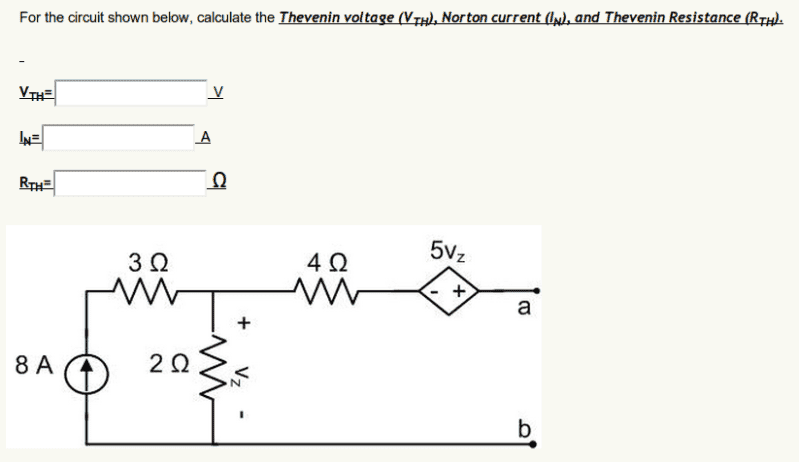# Thevenin w/ Dependent Sources

## Homework Statementv = IR

## The Attempt at a Solution

Okay, so up until this point, I was doing okay... but now I'm stuck.

I do not understand how I'm supposed to work with this because until now I have not had a dependent source.

Am I supposed to start by doing a KCL on the loop to the left?

Also, this may sound stupid, but on the resistor that is running in parallel, why is there a +/- Vz symbol? Is it just there to point direction of current flow or am I missing something?

Am I supposed to start by doing a KCL on the loop to the left?

Yes.

This always tripped me up too. You see, there's all this stuff on that top node and you are supposed to find the open circuit voltage? Geez. Why is all that stuff there, how can that not be a factor in the open circuit voltage?

So, your assumption is correct! Since the loop to the left is open, you just imagine that stuff is gone and you are just trying to find the voltage across that one resistor (Vz). Since there is a current source...I think you know what to do.

1st:
find the Th. equivalent for the 8A-3ohm-2ohm circuit.
2nd:
connect the equivalent in series with 4ohm-5Vz

You can Ignore everything in the branch from the node to port 'a' because current will not travel somewhere that will trap it. After, you analyze what you have left and see what you need. So you need Vth and Rth. To find Rth, zero out your INDEPENDANT SOURCES, then find the Req. Then Rth will equal Req. To find Vth dont assume it is = Vz. Why? because Vth is measured at the terminal 'a' to 'b'. We said there is no current through the branch from node to 'a' so there is no voltage drop through the 4ohm resistor BUT there is a voltage dependant voltage source so you will have to deal with that to find the Vth